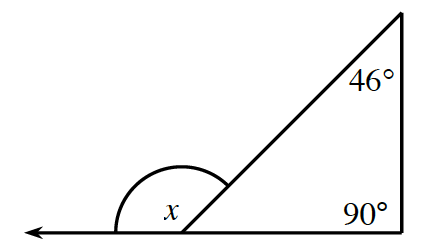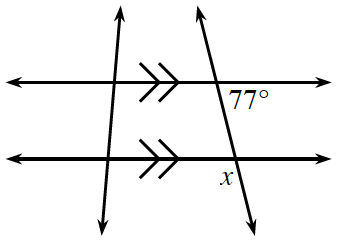### Home > GB8I > Chapter 9 Unit 3 > Lesson CC3: 9.2.2 > Problem9-79

9-79.

Calculate the value of $x$.

1.An exterior angle of a triangle is equal to the sum of the two remote interior angles.

$x = 46 + 90$

1.Notice that the $77º$ angle corresponds to the angle adjacent to angle $x$. This angle is complementary to angle $x$.

The vertical angle is equal to the $77º$ angle because they are alternate interior angles on parallel lines.